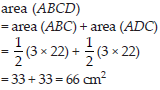# Perimeter and Area (Maths) Class 7 - NCERT Questions

Q 1.

The length and the breadth of a rectangular piece of land are 500 m and 300 m respectively. Find
(i) its area
(ii) the cost of the land, if 1 m2 of the land cost Rs. 10,000

SOLUTION: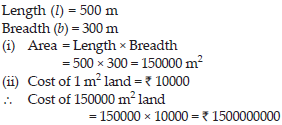Q 2.

Find the area of a square park whose perimeter is 320 m.

SOLUTION: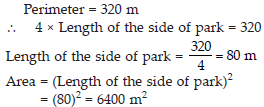Q 3.

Find the breadth of rectangular plot of land, if its area is 440 m2 and the length is 22 m. Also find its perimeter.

SOLUTION: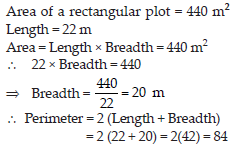Q 4.

The perimeter of a rectangular sheet is 100 cm. If the length is 35 cm, find its breadth. Also find the area.

SOLUTION: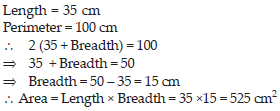Q 5.

The area of a square park is the same as of a rectangular park. If the side of the square park is 60 m and the length of the rectangular park is 90 m, find the breadth of the rectangular park.

SOLUTION: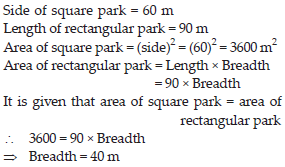Q 6.

A wire is in the shape of a rectangle. Its length is 40 cm and breadth is 22 cm. If the same wire is rebent in the shape of a square, what will be the measure of each side. Also find which shape encloses more area?

SOLUTION: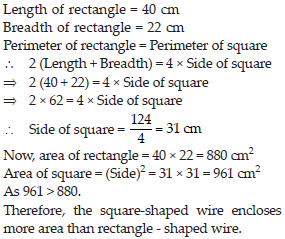Q 7.

The perimeter of a rectangle is 130 cm. If the breadth of the rectangle is 30 cm, find its length. Also find the area of the rectangle.

SOLUTION: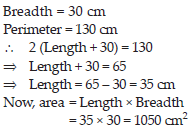Q 8.

A door of length 2 m and breadth 1 m is fitted in a wall. The length of the wall is 4.5 m and the breadth is 3.6 m (see the given figure). Find the cost of white washing the wall, if the rate of white washing the wall is Rs. 20 per m2.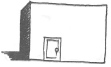SOLUTION: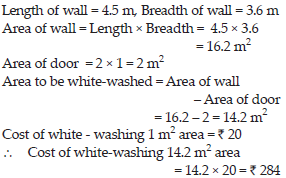Q 9.

Find the area of each of the following parallelograms: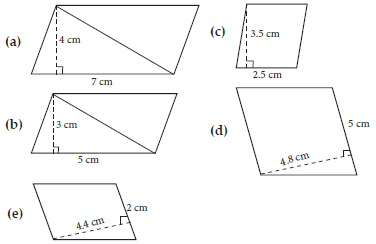SOLUTION: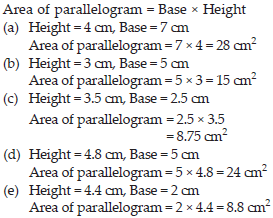Q 10.

Find the area of each of the following triangles: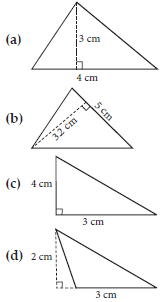SOLUTION: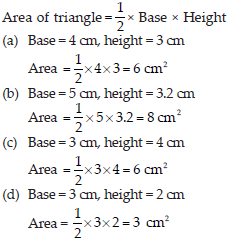Q 11.

Find the missing values: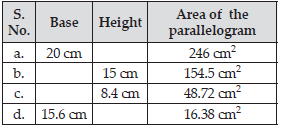SOLUTION: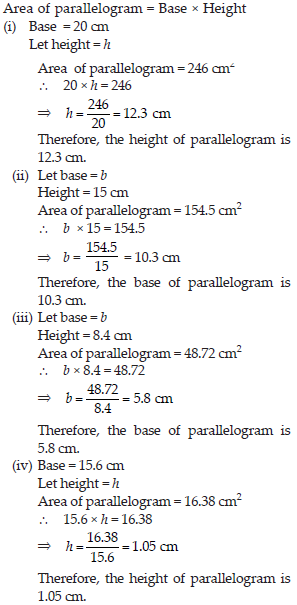Q 12.

Find the missing values: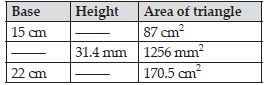SOLUTION: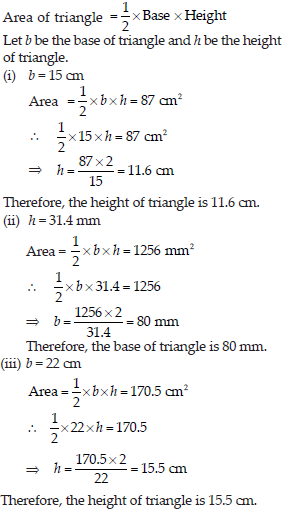Q 13.

PQRS is a parallelogram (see the given figure).QM is the height from Q to SR and QN is the height from Q to PS. If SR = 12 cm and QM = 7.6 cm. Find:
(i) the area of the parallelogram PQRS (ii) QN, if PS = 8 cm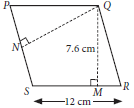SOLUTION: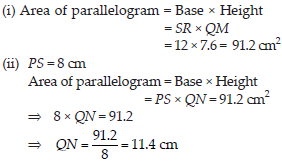Q 14.

DL and BM are the heights on sides AB and AD respectively of parallelogram ABCD (see the given figure).
If the area of the parallelogram is 1470 cm2, AB = 35 cm and AD = 49 cm, find the length of BM and DL.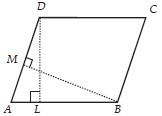SOLUTION: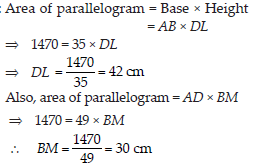Q 15.

∆ABC is right angled at A (see the given figure). AD is perpendicular to BC. If AB = 5 cm, BC = 13 cm and AC = 12cm, find the area of ∆ABC. Also find the length of AD.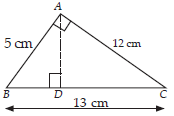SOLUTION: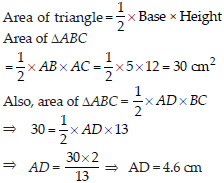Q 16.

∆ABC is isosceles with AB = AC = 7.5 cm and BC = 9 cm (see the given figure). The height AD from A to BC, is 6 cm. Find the area of ∆ABC. What will be the height from C to AB i.e., CE?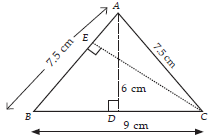SOLUTION: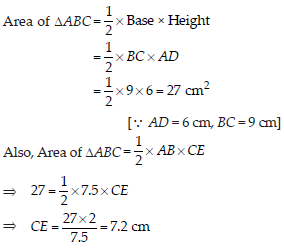Q 17.

Find the circumference of the circle with the following radius: (Take π = 22/7)
(A) 14 cm
(B) 28 mm
(C) 21 cm

SOLUTION: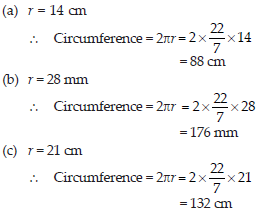Q 18.

Find the area of the following circles, given that: (Take π = 22/7)
(B) diameter = 49 m

SOLUTION: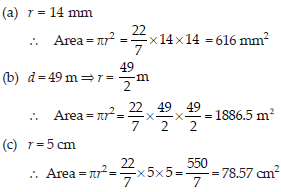Q 19.

If the circumference of circular sheet is 154 m, find its radius. Also find the area of the sheet. (Take π = 22/7)

SOLUTION: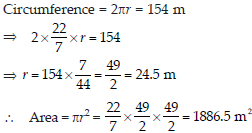Q 20.

A gardener wants to fence a circular garden of diameter 21 m. Find the length of the rope he needs to purchase, if he makes 2 rounds of fence. Also find the costs of the rope, if it cost Rs. 4 per meter. (take π = 22/7)

SOLUTION: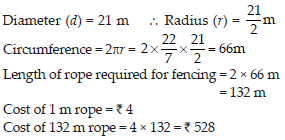Q 21.

From a circular sheet of radius 4 cm, a circle of radius 3 cm is removed. Find the area of the remaining sheet. (take π = 3.14)

SOLUTION: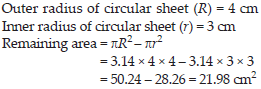Q 22.

Saima wants to put a lace on the edge of a circular table cover of diameter 1.5 m. Find the length of the lace required and also find its cost if one meter of the lace costs Rs. 15. (take π = 3.14)

SOLUTION: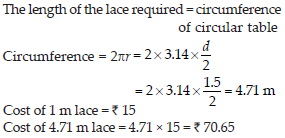Q 23.

Find the perimeter of the adjoining figure, which is a semicircle including its diameter.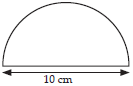SOLUTION: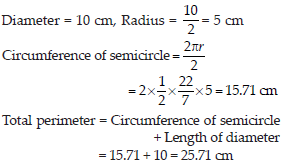Q 24.

Find the cost of polishing a circular table-top of diameter 1.6 m, if the rate of polishing is Rs. 15/m2. (take π = 3.14)

SOLUTION: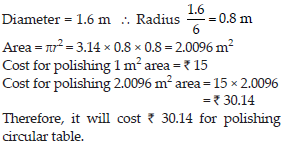Q 25.

Shazli took a wire of length 44 cm and bent it into the shape of a circle. Find the radius of that circle. Also find its area. If the same wire is bent into the shape of a square, what will be the length of each of its sides? Which figure encloses more area, the circle or the square? (take π = 22/7)

SOLUTION: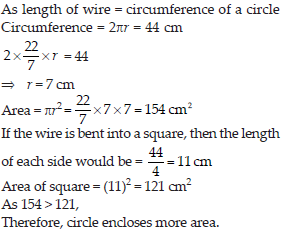Q 26.

From a circular card sheet of radius 14 cm, two circles of radius 3.5 cm and a rectangle of length 3 cm and breadth 1 cm are removed (as shown in the following figure.) Find the area of the remaining sheet. (take π = 22/7)SOLUTION: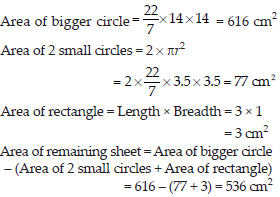Q 27.

A circle of radius 2 cm is cut out from a square piece of an aluminium sheet of side 6 cm. What is the area of the left over aluminium sheet? (take π = 3.14)

SOLUTION: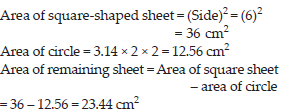Q 28.

The circumference of a circle is 31.4 cm. Find the radius and the area of the circle? (take π = 3.14)

SOLUTION: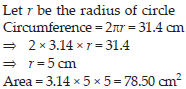Q 29.

A circular flower bed is surrounded by a path 4 m wide. The diameter of the flower bed is 66 m. What is the area of this path? (p = 3.14)

SOLUTION: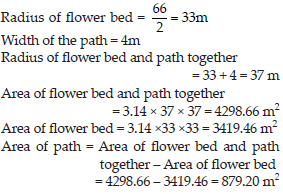Q 30.

A circular flower garden has an area of 314 m2. A sprinkler at the centre of the garden can cover an area that has a radius of 12 m. Will the sprinkler water the entire garden? (Take π = 3.14)

SOLUTION: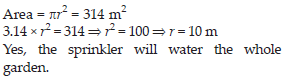Q 31.

Find the circumference of the inner and the outer circles, shown in the adjoining figure? (Take π = 3.14)

SOLUTION: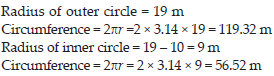Q 32.

How many times a wheel of radius 28 cm must rotate to go 352 m? (Take π = 22/7)

SOLUTION: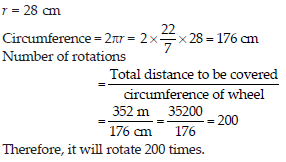Q 33.

The minute hand of a circular clock is 15 cm long. How far does the tip of the minute hand move in 1 hour? (Take π = 3.14)

SOLUTION: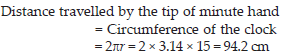Q 34.

A garden is 90 m long and 75 m broad. A path 5 m wide is to be built outside and around it. Find the area of the path. Also find the area of the garden in hectare.

SOLUTION: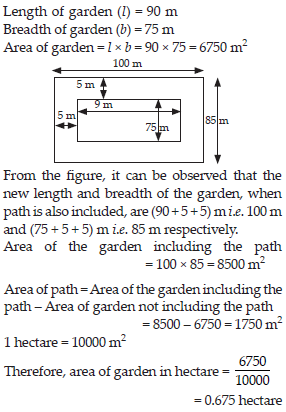Q 35.

A 3 m wide path runs outside and around a rectangular park of length 125 m and breadth 65 m. Find the area of the path.

SOLUTION: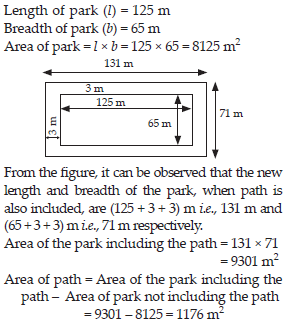Q 36.

A picture is painted on a cardboard 8 cm long and 5 cm wide such that there is a margin of 1.5 cm along each of its sides. Find the total area of the margin.

SOLUTION: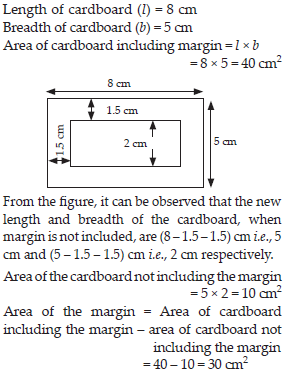Q 37.

A verandah of width 2.25 m is constructed all along outside a room which is 5.5 m long and 4 m wide. Find.
(i) the area of the verandah
(ii) the cost of cementing the floor of the verandah at the rate of Rs. 200 per m2

SOLUTION: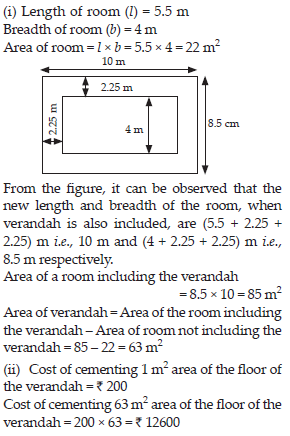Q 38.

A path 1 m wide is built along the border and inside a square garden of side 30 m. Find:
(i) the area of the path
(ii) the cost of planting grass in the remaining portion of the garden at the rate of Rs. 40 per m2.

SOLUTION: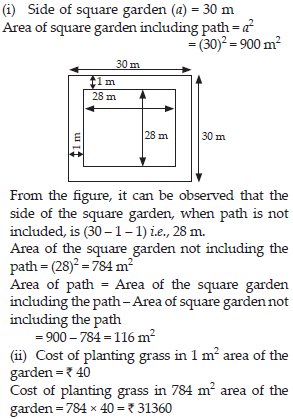Q 39.

Two cross roads, each of width 10 m, cut at right angles through the centre of a rectangular park of length 700 m and breadth 300 m and parallel to its sides. Find the area of the roads. Also find the area of the park excluding cross roads. Give the answer in hectares.

SOLUTION: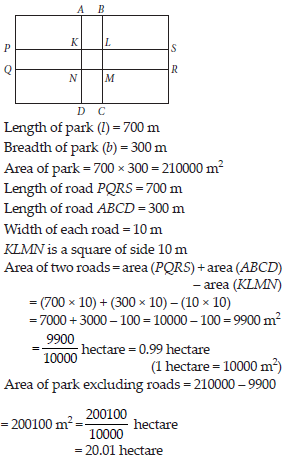Q 40.

Through a rectangular field of length 90 m and breadth 60 m, two roads are constructed which are parallel to the sides and cut each other at right angles through the centre of the fields. If the width of each road is 3 m, find
(i) the area covered by the roads.
(ii) the cost of constructing the roads at the rate of Rs. 110 per m2.

SOLUTION: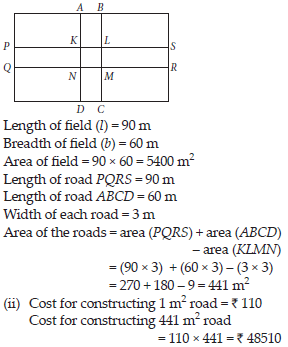Q 41.

Pragya wrapped a cord around a circular pipe of radius 4 cm (adjoining figure) and cut off the length required of the cord. Then she wrapped it around a square box of side 4 cm (also shown). Did she have any cord left? (π = 3.14)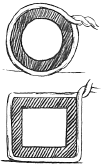SOLUTION: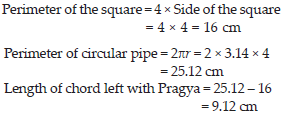Q 42.

The adjoining figure represents a rectangular lawn with a circular flower bed in the middle. Find :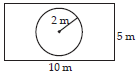(i) the area of the whole land
(ii) the area of the flower bed
(iii) the area of the lawn excluding the area of the flower bed
(iv) the circumference of the flower bed.

SOLUTION: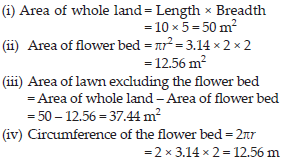Q 43.

In the following figures, find the area of the shaded portions: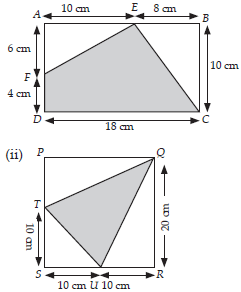SOLUTION: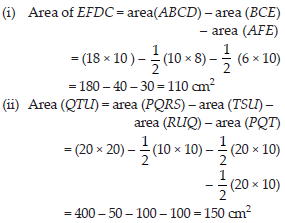Q 44.

Find the area of the quadrilateral ABCD. Here, AC = 22 cm, BM = 3 cm, DN = 3 cm, and BM ⊥ AC, DN ⊥ AC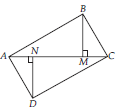SOLUTION: